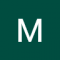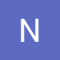Class Central is learner-supported. When you buy through links on our site, we may earn an affiliate commission.

# Mathematics for Machine Learning: Linear Algebra

## Overview

##### Class Central Tips
In this course on Linear Algebra we look at what linear algebra is and how it relates to vectors and matrices. Then we look through what vectors and matrices are and how to work with them, including the knotty problem of eigenvalues and eigenvectors, and how to use these to solve problems. Finally we look at how to use these to do fun things with datasets - like how to rotate images of faces and how to extract eigenvectors to look at how the Pagerank algorithm works.
Since we're aiming at data-driven applications, we'll be implementing some of these ideas in code, not just on pencil and paper. Towards the end of the course, you'll write code blocks and encounter Jupyter notebooks in Python, but don't worry, these will be quite short, focussed on the concepts, and will guide you through if you’ve not coded before.

At the end of this course you will have an intuitive understanding of vectors and matrices that will help you bridge the gap into linear algebra problems, and how to apply these concepts to machine learning.

## Syllabus

• Introduction to Linear Algebra and to Mathematics for Machine Learning
• In this first module we look at how linear algebra is relevant to machine learning and data science. Then we'll wind up the module with an initial introduction to vectors. Throughout, we're focussing on developing your mathematical intuition, not of crunching through algebra or doing long pen-and-paper examples. For many of these operations, there are callable functions in Python that can do the adding up - the point is to appreciate what they do and how they work so that, when things go wrong or there are special cases, you can understand why and what to do.
• Vectors are objects that move around space
• In this module, we look at operations we can do with vectors - finding the modulus (size), angle between vectors (dot or inner product) and projections of one vector onto another. We can then examine how the entries describing a vector will depend on what vectors we use to define the axes - the basis. That will then let us determine whether a proposed set of basis vectors are what's called 'linearly independent.' This will complete our examination of vectors, allowing us to move on to matrices in module 3 and then start to solve linear algebra problems.
• Matrices in Linear Algebra: Objects that operate on Vectors
• Now that we've looked at vectors, we can turn to matrices. First we look at how to use matrices as tools to solve linear algebra problems, and as objects that transform vectors. Then we look at how to solve systems of linear equations using matrices, which will then take us on to look at inverse matrices and determinants, and to think about what the determinant really is, intuitively speaking. Finally, we'll look at cases of special matrices that mean that the determinant is zero or where the matrix isn't invertible - cases where algorithms that need to invert a matrix will fail.
• Matrices make linear mappings
• In Module 4, we continue our discussion of matrices; first we think about how to code up matrix multiplication and matrix operations using the Einstein Summation Convention, which is a widely used notation in more advanced linear algebra courses. Then, we look at how matrices can transform a description of a vector from one basis (set of axes) to another. This will allow us to, for example, figure out how to apply a reflection to an image and manipulate images. We'll also look at how to construct a convenient basis vector set in order to do such transformations. Then, we'll write some code to do these transformations and apply this work computationally.
• Eigenvalues and Eigenvectors: Application to Data Problems
• Eigenvectors are particular vectors that are unrotated by a transformation matrix, and eigenvalues are the amount by which the eigenvectors are stretched. These special 'eigen-things' are very useful in linear algebra and will let us examine Google's famous PageRank algorithm for presenting web search results. Then we'll apply this in code, which will wrap up the course.

### Taught by

David Dye, Samuel J. Cooper and A. Freddie Page

## Reviews

3.1 rating, based on 8 reviews

Start your review of Mathematics for Machine Learning: Linear Algebra

•Maria Clarissa Fionalita
3
I feel like this course is underrated for people who want to learn machine learning. This, coming from someone who never did engineering degree. From the reviews seem like people were not satisfied with the lectures, but since week 1 they recommended...
•Naresh Sharma
1

Naresh Sharma completed this course.

The lectures on multi-variate calculus is too hand wavy and fast.. very difficult to follow.. as if the instructor is talking to himself. Probably not worth the effort.

Also only the first week is free... so the free thing is misleading.
• András Novoszáth completed this course, spending 5 hours a week on it and found the course difficulty to be easy.

I studied linear algebra at university, more than 10 years ago so I had some memories of the topic. I also familiar with python. I audited the course to gain practical experience and notation reading skills for my data science studies.

I liked the concepts and the lecture style. These were well done.

On the other hand they do not hand out almost any written material helping to understand the concepts and calculation required for the assessments. Accordingly, the assessments of the first week are quite easy but become much harder. Sadly not because the material is that complicated (what they explain, they demonstrate really well) but because they do not cover some parts of it.
20

Sagar Ladhwani completed this course and found the course difficulty to be very easy.

Although, pretty elementary (if you are an engineer), the course dives into the geometrical intuition of how vectors operate in space and the physical meaning of the various manipulations applied with matrices. The course material was quite easy to grasp and is for beginners in the area but if you opt for the 3 part specializations that this course belongs to, this one will help in establishing the footwork for the next courses.

• Ayse N.
20

Ayse N. is taking this course right now and found the course difficulty to be very hard.

I think the idea for the course is great. But I found the way they teach hard to follow. Just from the start, there are many questions that require prior understanding of Statistics, and the answers are not explained well. The content, as much as I have seen, is not very accessible.

Also for those who consider auditing this course; some videos are not available at all, so this course is not ideal for those who want to take it for free.
• Anonymous

Anonymous completed this course.

I speculate that the aims of the course were to simplify linear algebra to the bare essentials needed for machine learning. Unfortunately, the creators of the course have done it to an excessive extent such that many important concepts were glossed over, leaving the student extremely confused.

Would not recommend due to its hand-wavy nature. Take a proper linear algebra course if you can.
• Abdul Hannan
3

Abdul Hannan completed this course.

Its a great course for people trying to learn maths behind ML. I attended Prof Ng course on ML but thought I lack skills behind those algorithms. This course has given me lots of confidence to learn math behind ML. You have to put some efforts and search around the internet where things are not clear in the course.
• Benjamin Lau completed this course, spending 4 hours a week on it and found the course difficulty to be easy.

Break down all the essential knowledge required in the topic. I actually used this as an introductory course and delve deeper into each topics with other resources

###Never Stop Learning!

Get personalized course recommendations, track subjects and courses with reminders, and more.